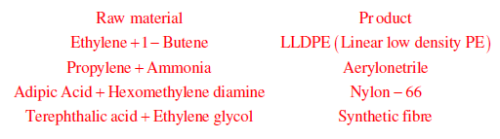Courses

# Chemical Engineering- CH 2014 GATE Paper (Practice Test)

## 65 Questions MCQ Test GATE Past Year Papers for Practice (All Branches) | Chemical Engineering- CH 2014 GATE Paper (Practice Test)

Description
This mock test of Chemical Engineering- CH 2014 GATE Paper (Practice Test) for GATE helps you for every GATE entrance exam. This contains 65 Multiple Choice Questions for GATE Chemical Engineering- CH 2014 GATE Paper (Practice Test) (mcq) to study with solutions a complete question bank. The solved questions answers in this Chemical Engineering- CH 2014 GATE Paper (Practice Test) quiz give you a good mix of easy questions and tough questions. GATE students definitely take this Chemical Engineering- CH 2014 GATE Paper (Practice Test) exercise for a better result in the exam. You can find other Chemical Engineering- CH 2014 GATE Paper (Practice Test) extra questions, long questions & short questions for GATE on EduRev as well by searching above.
QUESTION: 1

Solution:
QUESTION: 2

Solution:
QUESTION: 3

### Rajan was not happy that Sajan decided to do the project on his own. On observing his unhappiness, Sajan explained to Rajan that he preferred to work independently. Which one of the statements below is logically valid and can be inferred from the above sentences?

Solution:
QUESTION: 4

If y = 5x2 + 3, then the tangent at x = 0, y = 3

Solution: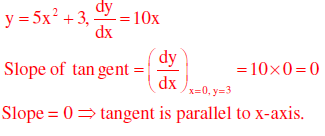*Answer can only contain numeric values
QUESTION: 5

A foundry has a fixed daily cost of Rs 50,000 whenever it operates and a variable cost of Rs
800Q, where Q is the daily production in tonnes. What is the cost of production in Rs per
tonne for a daily production of 100 tonnes?

Solution:

Fixed cost = Rs. 50,000
Variable cost = Rs. 800Q
Q = daily production in tones
For 100 tonnes of production daily, total cost of production
= 50,000+800×100 = 130,000
So, cost of production per tonne of daily production = 130000/100 = Rs. 1300

QUESTION: 6

Find the odd one in the following group: ALRVX, EPVZB, ITZDF, OYEIK

Solution:

ALRVX→only one vowel
EPVZB→only one vowel
ITZDF→only one vowel
OYEIK→three vowels

QUESTION: 7

Anuj, Bhola, Chandan, Dilip, Eswar and Faisal live on different floors in a six-storeyed
building (the ground floor is numbered 1, the floor above it 2, and so on). Anuj lives on an
even-numbered floor. Bhola does not live on an odd numbered floor. Chandan does not live
on any of the floors below Faisal’s floor. Dilip does not live on floor number 2. Eswar does
not live on a floor immediately above or immediately below Bhola. Faisal lives three floors
above Dilip. Which of the following floor-person combinations is correct?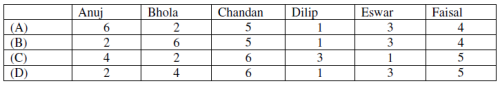Solution:

(a) Anuj: Even numbered floor (2,4,6)
(b) Bhola: Even numbered floor (2,4,6)
(c) Chandan lives on the floor above that of Faisal.
(d) Dilip: not on 2nd floor.
(e) Eswar: does not live immediately above or immediately below Bhola
From the options its clear, that only option (B) satisfies condition (e).
So, correct Ans is (B).

*Answer can only contain numeric values
QUESTION: 8

The smallest angle of a triangle is equal to two thirds of the smallest angle of a quadrilateral.
The ratio between the angles of the quadrilateral is 3:4:5:6. The largest angle of the triangle is
twice its smallest angle. What is the sum, in degrees, of the second largest angle of the
triangle and the largest angle of the quadrilateral?

Solution:

Let the angles of quadrilateral are 3x, 4x, 5x, 6x
So, 3x+4x+5x+6x = 360
x = 20
Smallest angle of quadrilateral = 3×20 = 60°
Smallest angle of triangle = 2/3 x 60o = 40o
Largest angle of triangle = 2×40° = 60°
Three angles of triangle are 40°, 60°, 80°
Largest angle of quadrilateral is 120°
Sum (2nd largest angle of triangle + largest angle of quadrilateral)
= 60°+120°=180°.

QUESTION: 9

One percent of the people of country X are taller than 6 ft. Two percent of the people of
country Y are taller than 6 ft. There are thrice as many people in country X as in country Y.
Taking both countries together, what is the percentage of people taller than 6 ft?

Solution:

Let number of people in country y = 100
So, number of people in country x = 300
Total number of people taller than 6ft in both the countries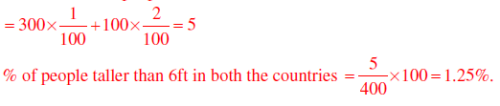QUESTION: 10

The monthly rainfall chart based on 50 years of rainfall in Agra is shown in the following
figure. Which of the following are true? (k percentile is the value such that k percent of the
data fall below that value)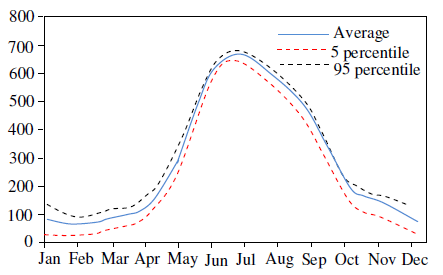(i) On average, it rains more in July than in December
(ii) Every year, the amount of rainfall in August is more than that in January
(iii) July rainfall can be estimated with better confidence than February rainfall
(iv) In August, there is at least 500 mm of rainfall

Solution:

In the question the monthly average rainfall chart for 50 years has been given.
Let us check the options.
(i) On average, it rains more in July than in December  correct.
(ii) Every year, the amount of rainfall in August is more than that in January.

⇒ may not be correct because average rainfall is given in the question.

(iii) July rainfall can be estimated with better confidence than February rainfall.

⇒ From chart it is clear the gap between 5 percentile and 95 percentile from average is higher in February than that in July  ⇒ correct.
(iv) In August at least 500 mm rainfall

 ⇒May not be correct, because its 50 year average.
So correct option (B) (i) and (iii).

QUESTION: 11

Q.No-11-35 Carry One Mark Each

Q.

Gradient of a scalar variable is always

Solution: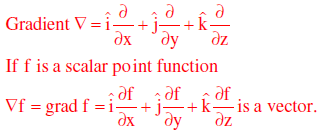QUESTION: 12

For the time domain function, f(t) = t2 ,which ONE of the following is the Laplace transform
of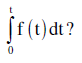Solution: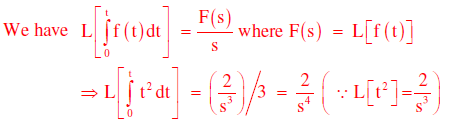QUESTION: 13

If f*(x) is the complex conjugate of f(x) = cos(x) + i sin(x), then for real a and b,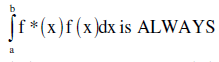Solution: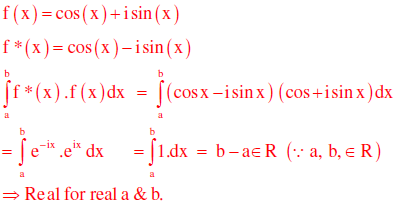QUESTION: 14

If f(x) is a real and continuous function of x, the Taylor series expansion of f(x) about
its minima will NEVER have a term containing

Solution: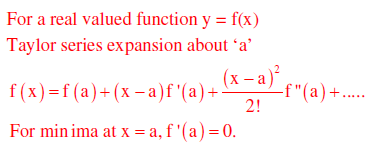So, Taylor series expansion of f(x) about ‘a’ will never contain first derivative term.

QUESTION: 15

From the following list, identify the properties which are equal in both vapour and
liquid phases at equilibrium
P. Density                                         Temperature
R. Chemical potential                    S. Enthalpy

Solution: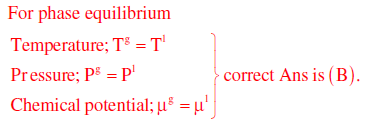QUESTION: 16

In a closed system, the isentropic expansion of an ideal gas with constant specific heats is
represented by

Solution: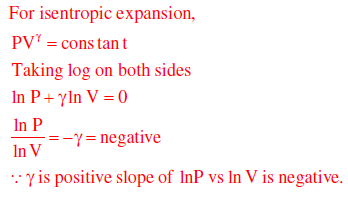QUESTION: 17

Match the following :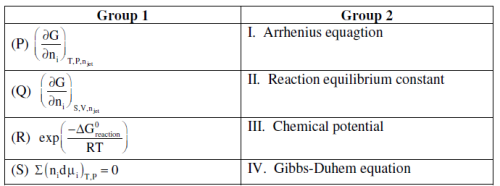Solution:
QUESTION: 18

In order to achieve the same conversion under identical reaction conditions and feed flow rate
for a non-autocatalytic reaction of positive order, the volume of an ideal CSTR is

Solution: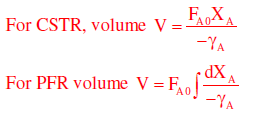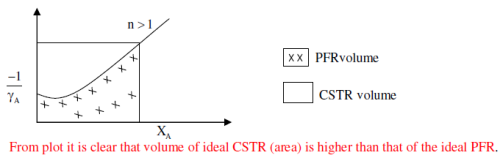QUESTION: 19

Integral of the time-weighted absolute error (ITAE) is expressed as

Solution:

Let number of people in country y = 100
So, number of people in country x = 300
Total number of people taller than 6ft in both the countries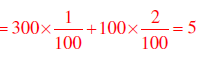% of people taller than 6ft in both the countries =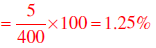QUESTION: 20

A unit IMPULSE response of a first order system with time constant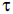and steady
state gain Kp is given by

Solution: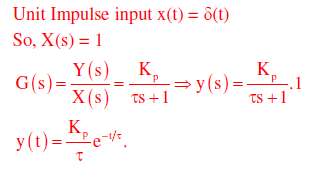QUESTION: 21

In a completely opaque medium, if 50% of the incident monochromatic radiation is absorbed,
then which of the following statements are CORRECT?
(P) 50% of the incident radiation is reflected
(Q) 25% of the incident radiation is reflected
(R) 25% of the incident radiation is transmitted
(S) No incident radiation is transmitted

Solution: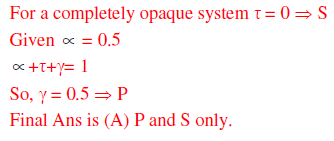QUESTION: 22

In case of a pressure driven laminar flow of a Newtonian fluid of viscosity (μ) through a
horizontal circular pipe, the velocity of the fluid is proportional to

Solution: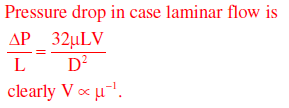QUESTION: 23

Which of the following statements are CORRECT?
(P) For a rheopectic fluid, the apparent viscosity increases with time under a constant applied
shear stress
(Q) For a pseudoplastic fluid, the apparent viscosity decreases with time under a constant
applied shear stress
(R) For a Bingham plastic, the apparent viscosity increases exponentially with the
deformation rate
(S) For a dilatant fluid, the apparent viscosity increases with increasing deformation rate

Solution:
QUESTION: 24

Assume that an ordinary mercury-in-glass thermometer follows first order dynamics with a
time constant of 10s. It is at a steady state temperature of 0°C. At time t = 0, the thermometer
is suddenly immersed in a constant temperature bath at 100°C. The time required (in s) for
the thermometer to read 95°C, approximately is

Solution: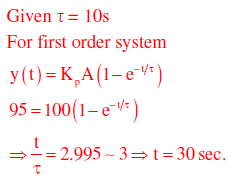QUESTION: 25

Packed towers are preferred for gas-liquid mass transfer operations with foaming liquids
because

Solution:
QUESTION: 26

A spherical storage vessel is quarter–filled with toluene. The diameter of the vent at the top of
the vessel is 1/20th of the diameter of the vessel. Under the steady state condition, the
diffusive flux of toluene is maximum at

Solution:

Diffusive flux is maximum at the vent and remains same throughout the vent line at steady state.

QUESTION: 27

In order to produce fine solid particles between 5 and 10 μm, the appropriate size reducing
equipment is

Solution:
QUESTION: 28

Slurries are most conveniently pumped by a

Solution:
QUESTION: 29

Assuming the mass transfer coefficients in the gas and the liquid phases are comparable, the
absorption of CO2 from reformer gas (CO2+H2) into an aqueous solution of diethanolamine is
controlled by

Solution:
QUESTION: 30

Which ONE of the following statements is CORRECT for the surface renewal theory?

Solution:
QUESTION: 31

Steam economy of a multiple effect evaporator system is defined as

Solution:
QUESTION: 32

Decomposition efficiency (D) of an electrolytic cell used for producing NaOH is defined as

Solution:
QUESTION: 33

The vessel dispersion number for an ideal CSTR is

Solution: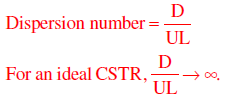QUESTION: 34

Catalytic cracking is

Solution:
QUESTION: 35

Which ONE of the following statements is CORRECT?

Solution:
*Answer can only contain numeric values
QUESTION: 36

Q.No-36-65 Carry Two Marks Each

Q.

Consider the following differential equation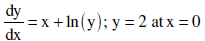The solution of this equation at x = 0.4 using Euler method with a step size of h= 0.2 is

Solution: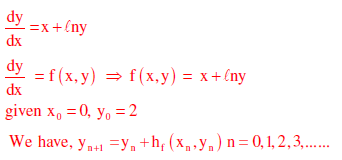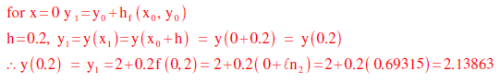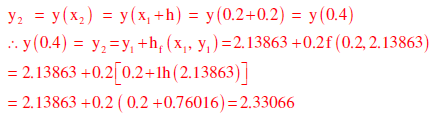QUESTION: 37

The integrating factor for the differential equation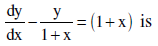Solution:

Given differential equation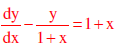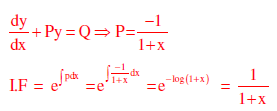QUESTION: 38

The differential equation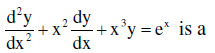Solution: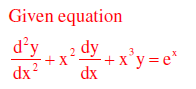This is clearly a linear differential equation
Order = 2
Degree = 1.

QUESTION: 39

Consider the following two normal distributions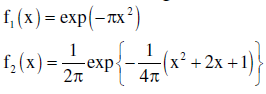If μ and σ denote the mean and standard deviation, respectively, then

Solution: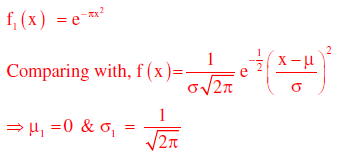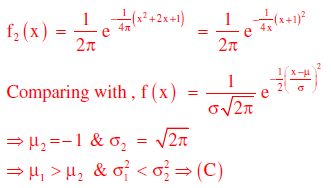*Answer can only contain numeric values
QUESTION: 40

In rolling of two fair dice, the outcome of an experiment is considered to be the sum of the
numbers appearing on the dice. The probability is highest for the outcome of ___________

Solution: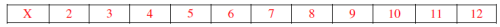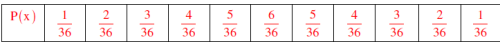Where X is a random variable denotes the sum of the numbers appearing on the dice.
P(x) = corresponding probabilities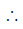The probability is highest for the outcome “7” i.e., 6/36

QUESTION: 41

A spherical ball of benzoic acid (diameter = 1.5 cm) is submerged in a pool of still water. The solubility and diffusivity of benzoic acid in water are 0.03 kmol/m3 and 1.25 x 10-9 m2/s respectively. Sherwood number is given as Sh = 2.0 + 0.6 0.33 Re0.5 Sc0.33 . The initial rate of dissolution (in kmol/s) of benzoic acid approximately is

Solution: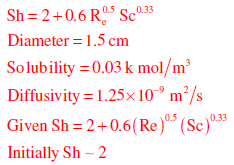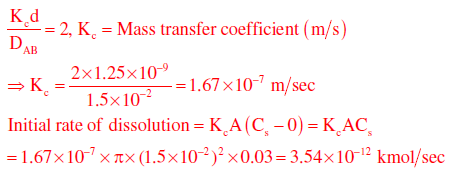*Answer can only contain numeric values
QUESTION: 42

A wet solid of 100 kg is dried from a moisture content of 40wt% to 10wt%. The critical moisture content is 15wt% and the equilibrium moisture content is negligible. All moisture contents are on dry basis. The falling rate is considered to be linear. It takes 5 hours to dry the material in the constant rate period. The duration (in hours) of the falling rate period is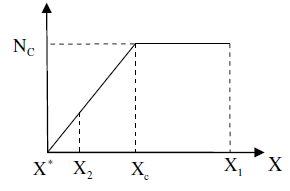Solution:

Given X1 = 0.4
X2 = 0.10
XC = 0.15
X* = 0
Constant rate period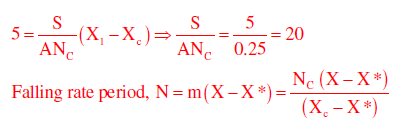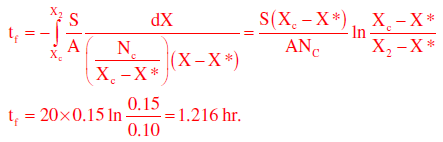*Answer can only contain numeric values
QUESTION: 43

A brick wall of 20 cm thickness has thermal conductivity of 0.7 W m-1 K-1. An insulation of thermal conductivity 0.2 W m-1 K-1 is to be applied on one side of the wall, so that the heat transfer through the wall is reduced by 75%. The same temperature difference is maintained across the wall before and after applying the insulation. The required thickness (in cm) of the insulation is ____________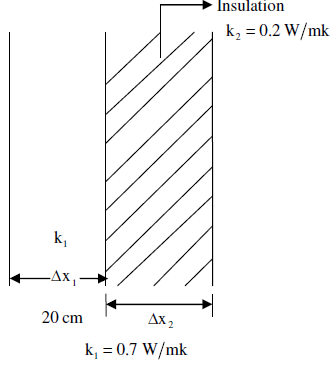Solution: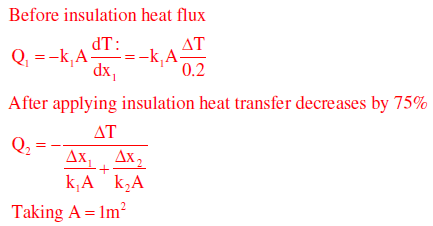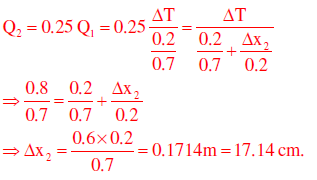*Answer can only contain numeric values
QUESTION: 44

An oil with a flow rate of 1000 kg/h is to be cooled using water in a double-pipe counter-flow heat exchanger from a temperature of 70°C to 40°C. Water enters the exchanger at 25°C and leaves at 40°C. The specific heats of oil and water are 2 kJ kg-1 K-1 and 4.2 kJ kg-1 K-1, respectively. The overall heat transfer coefficient is 0.2 kW m-2 K-1. The minimum heat exchanger area (in m2) required for this operation is ______________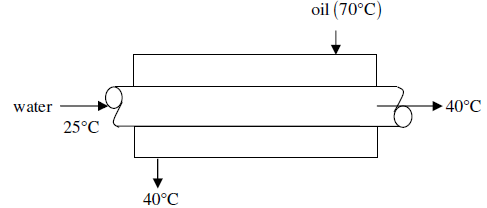Solution:

Given Cpo =2 kJkg-1 K-1

Cpo =4.2 kJkg-1 K-1

U= 0.2kW m-2K-1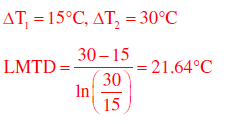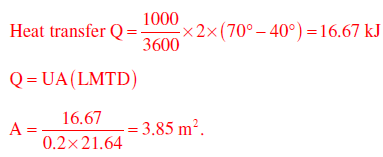QUESTION: 45

Which ONE of the following is CORRECT for an ideal gas in a closed system?

Solution: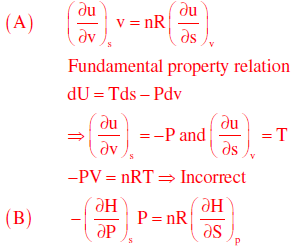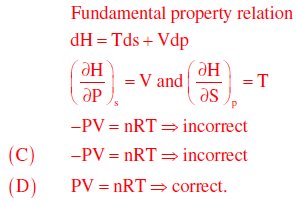*Answer can only contain numeric values
QUESTION: 46

A binary distillation column is operating with a mixed feed containing 20 mol% vapour. If
the feed quality is changed to 80 mol% vapour, the change in the slope of the q-line is

Solution: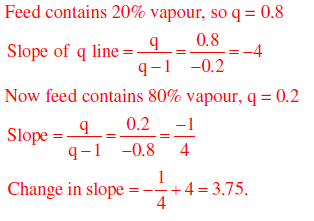QUESTION: 47

A homogeneous reaction (R → P) occurs in a batch reactor. The conversion of the reactant R is 67% after 10 minutes and 80% after 20 minutes. The rate equation for this reaction is

Solution: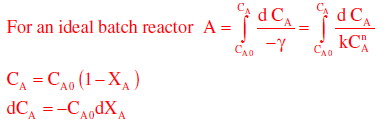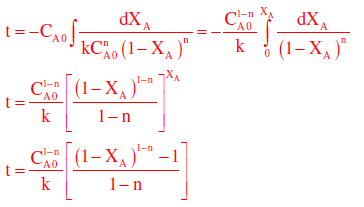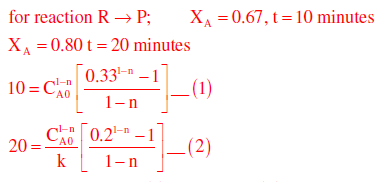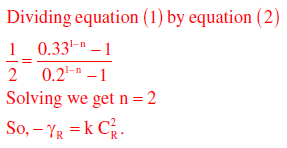QUESTION: 48

A vapour phase catalytic reaction (Q + R →S) follows Rideal mechanism (R and S are not adsorbed). Initially, the mixture contains only the reactants in equimolar ratio. The surface reaction step is rate controlling. With constants a and b, the initial rate of reaction ( −r0 ) in terms of total pressure (PT) is given by

Solution: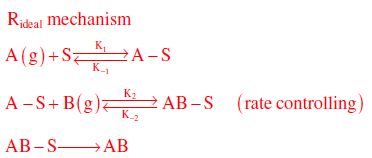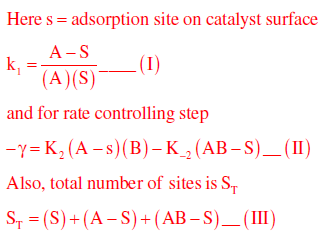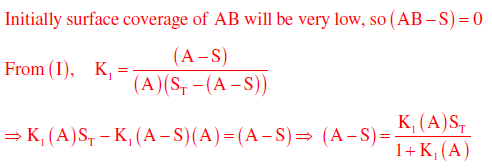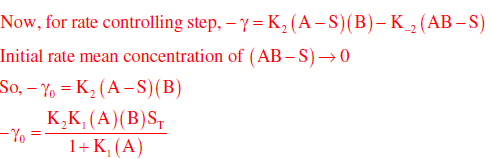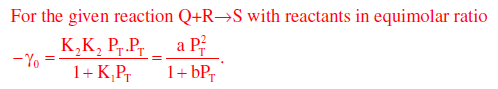*Answer can only contain numeric values
QUESTION: 49

A incompressible fluid is flowing through a contraction section of length L and has a 1-D (x
direction) steady state velocity distribution,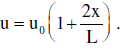If u0 = 2m/ s and L = 3m, the
convective acceleration (in m/s2) of the fluid at L is _______

Solution: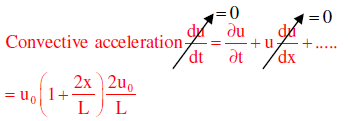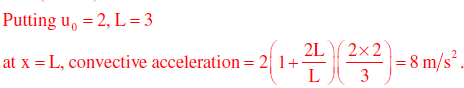QUESTION: 50

Match the following: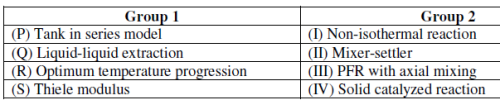Solution:

Tank in series model → PFR with axial mixing
Liquid-liquid extraction → Mixer settler
Optimum temperature progression → Non-isothermal reaction
Thiele modulus → Solid catalyst reaction.

*Answer can only contain numeric values
QUESTION: 51

Two elemental gases (A and B) are reacting to form a liquid (C) in a steady state process as per the reaction. A + B→C. The single-pass conversion of the reaction is only 20% and hence recycle is used. The product is separated completely in pure form. The fresh feed has 49 mol% of A and B each along with 2 mol% impurities. The maximum allowable impurities in the recycle stream is 20 mol%. The amount of purge stream (in moles) per 100 moles of the fresh feed is ___________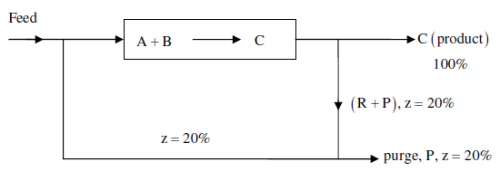Solution:

Basis = 100 moles of fresh feed
A = 49 moles; B = 49 moles;
Inert, z = 2 moles
Overall balance on inert

2 = P×0.2 ⇒ P = 10

*Answer can only contain numeric values
QUESTION: 52

Carbon monoxide (CO) is burnt in presence of 200% excess pure oxygen and the flame
temperature achieved is 2298 K. The inlet streams are at 25 °C. The standard heat of
formation (at 25 °C) of CO and CO2 are -110kJ mol-1 and -390kJ mol-1, respectively. The
heat capacities (in J mol-1 K-1) of the components are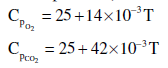where, T is the temperature in K. The heat loss (in kJ) per mole of CO burnt
is_____________

Solution: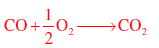Basis: 1 mole of CO burnt
O2 supplied = 0.5×3 = 1.5 mole
Unreacted O2 in product = 1 mole
Standard heat of reaction = - 390 – (-110) = - 280 kJ/mol.
Heat of reactants = 0 (at 298k)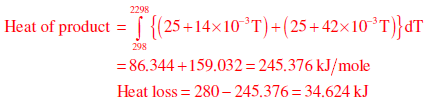*Answer can only contain numeric values
QUESTION: 53

A cash flow of Rs. 12,000 per year is received at the end of each year (uniform periodic
payment) for 7 consecutive years. The rate of interest is 9% per year compounded annually.
The present worth (in Rs.) of such cash flow at time zero is __________

Solution: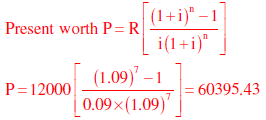*Answer can only contain numeric values
QUESTION: 54

A polymer plant with a production capacity of 10,000 tons per year has an overall yield of 70%, on mass basis (kg of product per kg of raw material). The raw material costs Rs. 50,000 per ton. A process modification is proposed to increase the overall yield to 75% with an investment of Rs. 12.5 crore. In how many years can the invested amount be recovered with the additional profit? ___________

Solution: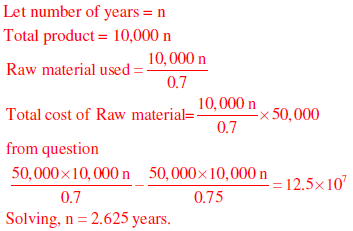*Answer can only contain numeric values
QUESTION: 55

A step change of magnitude 2 is introduced into a system having the following transfer
function :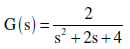The percent overshoot is ___________________.

Solution: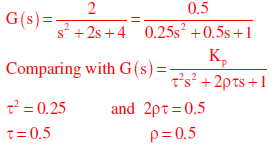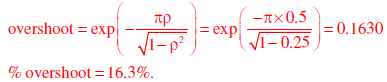QUESTION: 56

Given below is a simplified block diagram of a feedforward control system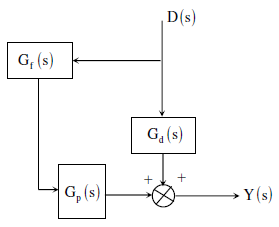The transfer function of the process is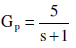and the disturbance transfer function is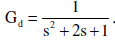The transfer function of the PERFECT feed forward controller, Gf(s) is

Solution: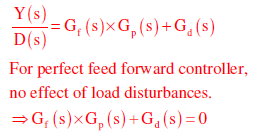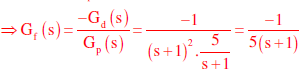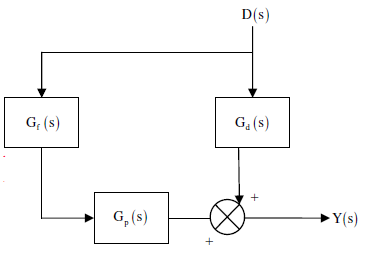*Answer can only contain numeric values
QUESTION: 57

The bottom face of a horizontal slab of thickness 6 mm is maintained at 300oC. The top face is exposed to a flowing gas at 30oC. The thermal conductivity of the slab is 1.5 W m-1 K-1 and the convective heat transfer coefficient is 30 W m-2 K-1. At steady state, the temperature (in oC) of the top face is __________ .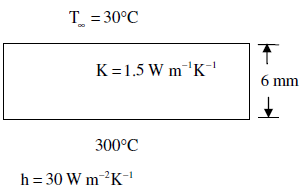Solution:

At steady state
heat flux due to conduction = heat flux due to convection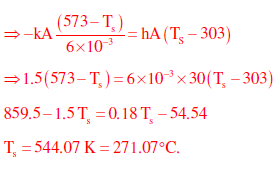*Answer can only contain numeric values
QUESTION: 58

In a steady incompressible flow, the velocity distribution is given by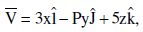where, V is in m/s and x, y, and z are in m. In order to satisfy the mass conservation, the value
of the constant P (in s-1) is ____________.

Solution: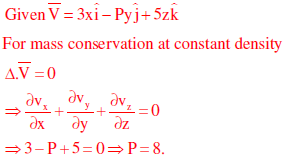QUESTION: 59

Match the following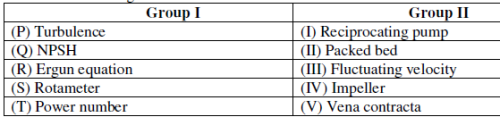Solution:

Turbulence: Characterized by fluctuating velocity.
Ergun equation: for calculating pressure drop in packed bed.
Power number: For calculating power consumption in mixing tank.

*Answer can only contain numeric values
QUESTION: 60

In a steady and incompressible flow of a fluid (density = 1.25 kg m-3), the difference between stagnation and static pressures at the same location in the flow is 30 mm of mercury (density = 13600 kg m-3). Considering gravitational acceleration as 10 m s-2, the fluid speed (in m s-1) is ______________

Solution: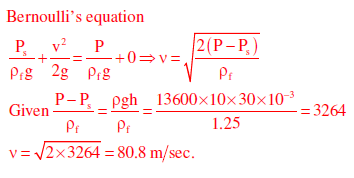*Answer can only contain numeric values
QUESTION: 61

Consider a binary liquid mixture at equilibrium with its vapour at 25OC. Antoine equation for this system is given as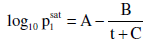where t is in OC and p in Torr.
The Antoine constants (A, B, and C) for the system are given in the following table.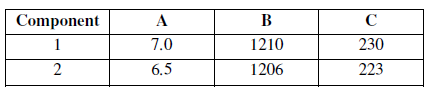The vapour phase is assumed to be ideal and the activity coefficients ( γi)  for the non-ideal
liquid phase are given by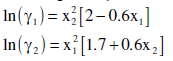If the mole fraction of component 1 in liquid phase (x1) is 0.11, then the mole fraction of
component 1 in vapour phase (y1) is ___________

Solution: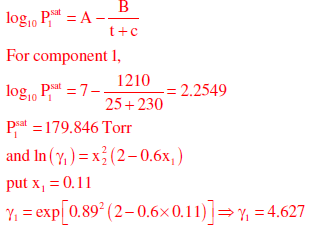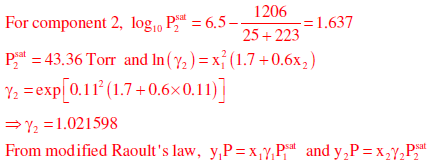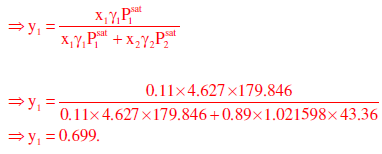QUESTION: 62

A process with transfer function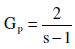is to be controlled by a feedback proportional controller with a gain Kc. If the transfer functions of all other elements in the control loop are unity, then which ONE of the following conditions produces a stable closed loop response?

Solution: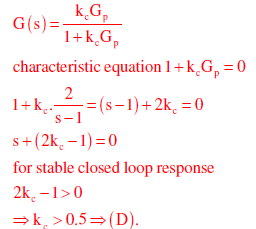*Answer can only contain numeric values
QUESTION: 63

Consider the following block diagram for a closed-loop feedback control system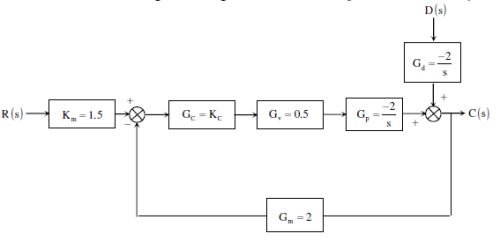A proportional controller is being used with KC = −4 . If a step change in disturbance of magnitude 2 affects the system, then the value of the offset is ____________

Solution: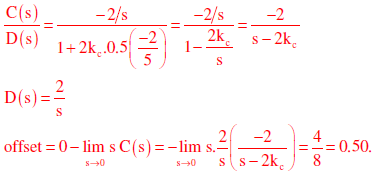QUESTION: 64

Determine the correctness or otherwise of the following Assertion [a] and Reason [r].
Assertion: Significant combustion of coke takes place only if it is heated at higher
temperature in presence of air.

Reason: C + O2 →CO2 is an exothermic reaction

Solution:

Both [a] and [r] are true but [r] is not the correct reason for [a].

QUESTION: 65

Match the raw materials of Groups 1 and 2 with the final products of Group 3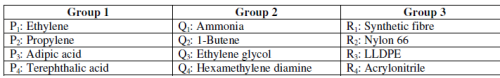Solution: